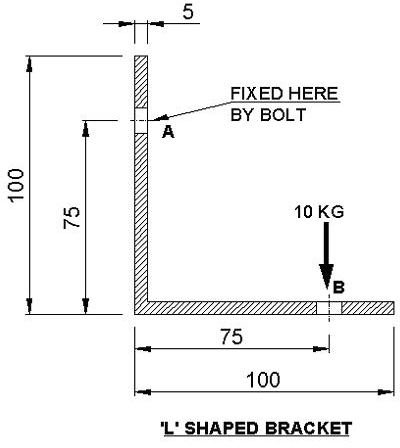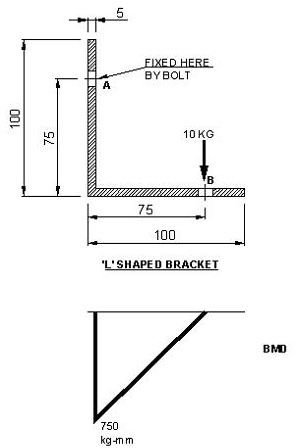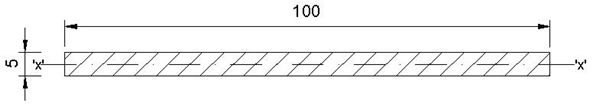# Mechanical Design Tutorials on Basic calculationsToday I will talk about design of a simple “L” shaped bracket, one side of it is clamped and at the other side it is carrying some amount of load.

In the picture above the bracket is subjected to a load of 10 kg at point ‘B’ and fixed by bolt at point ‘A’. For initial design we have started with 5 mm thick plate.

The following steps need to be followed for finding out the actual thickness needed for the bracket:

1. Calculating maximum bending moment: In order to find out the section where the maximum bending stress occurs, we need to draw bending moment diagram.

Let’s consider our case as a cantilever beam (though it is not a proper cantilever beam but for the initial analysis we are considering this as a cantilever beam).From the bending moment diagram we can easily point out the location of maximum bending moment and the value of the maximum bending moment is 750 kg-mm.

So, the maximum bending moment M=750 kg-mmThe area moment of inertia for this section = (bd^3)/12

= 1041.667 mm4

3. Calculating bending stress: The classic formula for determining the bending stress in a member is:

f/y=M/ I

• f is the bending stress
• M – the moment at the neutral axis
• y – the perpendicular distance to the neutral axis
• Ix – the area moment of inertia about the neutral axis x
• b – the width of the section being analyzed
• h – the depth of the section being analyzed

f = M.y/ I

= 750 X 2.5/1041.667 kg/mm2

= 1.8 kg/mm2

If we consider a steel with yield strength is 25 kg/mm2, then we can see that stress generated in the clip is substantially low , so we have the chance to further reduce the thickness of the clip.

Though this is a very simple example of calculations but if we follow this methodology for new concept design can initially decide the size and start modelling with that size then the analysis iterations also could be reduced significantly, and so the overall design time and cost.